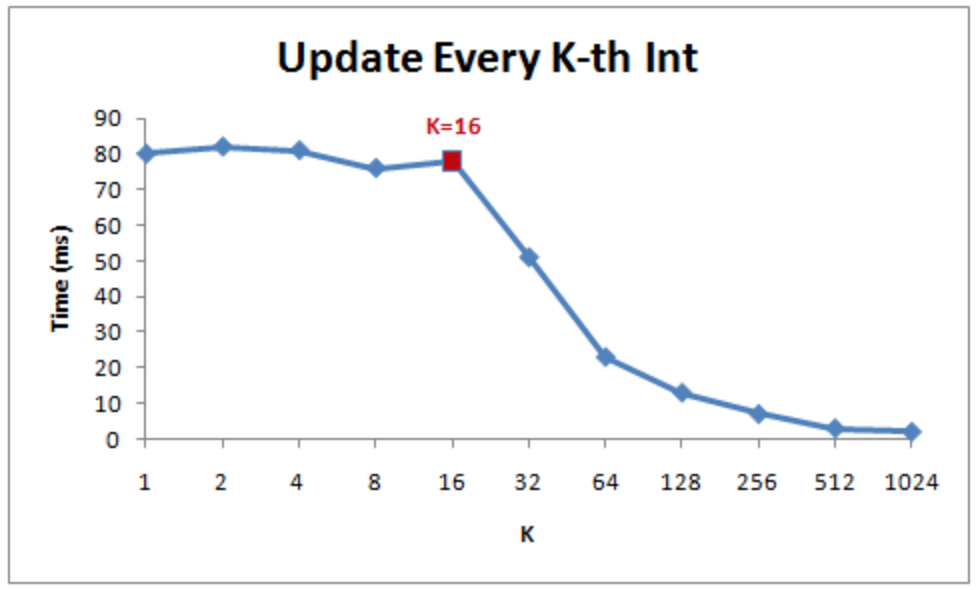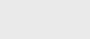2年前 (2022-02-09) |   抢沙发  268

[收起] 文章目录

Joshua Bloch 在推特上发了一个链接（http://igoro.com/archive/gallery-of-processor-cache-effects/），使用高级编程语言（如C#）演示了CPU缓存的效果。强烈推荐！## 当心局部优化

Igor Ostrovsky以一个简单的问题开始他的文章：以下哪种方法运行得更快？

``````public class CacheLines {
private static int[] array = new int[64 * 1024 * 10];

private static void loop1() {
int length = array.length;
for (int i = 0; i < length; i=i+1)
array[i] --;
}

private static void loop2() {
int length = array.length;
for (int i = 0; i < length; i += 2)
array[i] --;
}
}``````

``````Step   1/10000 took 1250.627 ms  that's  100% of the expected time
Step   2/10000 took 1668.458 ms  that's  266% of the expected time
Step   4/10000 took 1159.842 ms  that's  370% of the expected time
Step   8/10000 took 1039.095 ms  that's  664% of the expected time
Step  16/10000 took 1022.674 ms  that's 1308% of the expected time
Step  32/10000 took  530.95  ms  that's 1358% of the expected time
Step  64/10000 took  272.518 ms  that's 1394% of the expected time
Step 128/10000 took  138.968 ms  that's 1422% of the expected time
Step 256/10000 took  100.921 ms  that's 2065% of the expected time
``````

## 检查汇编代码

JVM的一个更有趣、也是相当未知的特性是，您可以看到优化器在工作，一直到机器代码。添加JVM选项`-XX:+UnlockDiagnosticVMOptions -XX:+PrintAssembly`会输出您该方法的源代码：

``````0x00000000027f0f69: dec     dword ptr [r10+r13*4+10h]
;*iastore
; - CacheLines::loop1@18 (line 199)
0x00000000027f0f6e: inc     r13d              ;*iinc
; - CacheLines::loop1@19 (line 198)
0x00000000027f0f71: cmp     r13d,r9d
0x00000000027f0f74: jl      27f0f69h          ;*if_icmplt
; - CacheLines::loop1@24 (line 198)``````

``````for (int i = 0; i < length; i += 2)
array[i] --;
``````

1. `dec dword ptr [r10+r13*4+10h]`这是最复杂的线路，也是最让我惊讶的线路。整个行数组[i]——可以用一个操作码来表示。r10+10h是指向字节数组的指针，r13*4是数组内的偏移量。按照惯例，方括号指的是由括号内的数字指示的存储单元。所以dec[r10+r13+10h]取整数数组的一个值，将其递减，并将其写回内存。

2. inc r13d递增循环计数器（变量i）。

3. cmp r13d、r9d检查是否已到达回路末端。

4. jl 27f0f69h是一个有条件的转到。根据之前的检查，指令指针被设置回四条指令，再次运行循环。

`loop2（）`的运行速度比`loop1（）`慢了一点，所以我们也应该检查机器代码。也许有一个奇怪的优化？

``````0x00000000027f1508: dec     dword ptr [r11+rbx*4+10h]
;*iastore
; - CacheLines::loop2@18 (line 205)
; - CacheLines::loop2@19 (line 204)
0x00000000027f1510: cmp     ebx,r10d
0x00000000027f1513: jl      27f1508h          ;*if_icmplt
; - CacheLines::loop2@24 (line 204)``````

## step 计数

``````private static void testVariableStep(final int step) {
long start3 = System.nanoTime();
loopVariableStep(step);
long time3 = System.nanoTime() - start3;
cumulated3 += time3;
}
private static void loopVariableStep(final int step) {
int length = array.length;
for (int i = 0; i < length; i += step)
array[i]--;
}``````

``````Step   1/10000 took 5363.778 ms  that's 100% of the expected time
Step   2/10000 took 2703.319 ms  that's 100% of the expected time
Step   4/10000 took 1428.127 ms  that's 106% of the expected time
Step   8/10000 took 1024.124 ms  that's 152% of the expected time
Step  16/10000 took  985.169 ms  that's 293% of the expected time
Step  32/10000 took  492.46  ms  that's 293% of the expected time
Step  64/10000 took  250.679 ms  that's 299% of the expected time
Step 128/10000 took  122.265 ms  that's 291% of the expected time
Step 256/10000 took   69.627 ms  that's 332% of the expected time
``````

``````0x00000000027404b0: cmp     r9d,r10d
; - CacheLines::loopVariableStep@15 (line 295)
0x00000000027404b5: dec     dword ptr [r11+r9*4+10h]
;*if_icmplt
; - CacheLines::loopVariableStep@25 (line 294)
0x00000000027404ba: add     r9d,edx           ; OopMap{r11=Oop rbp=NarrowOop off=61}
;*if_icmplt
; - CacheLines::loopVariableStep@25 (line 294)
0x00000000027404bd: test    dword ptr [120000h],eax  ;   {poll}
0x00000000027404c3: cmp     r9d,r10d
0x00000000027404c6: jl      27404b0h          ;*synchronization entry
; - CacheLines::loopVariableStep@-1 (line 293)
``````

• 比较`i < length`执行两次（cmp r9、r10d，然后在第1-2行和第11-12行进行条件跳转）。如果需要，第一次检查会引发`IndexOutOfBoundsException`。重复的支票并不能解释差异。它只增加了几个CPU周期。先进的CPU技术，如无序执行和分支预测，可以几乎完全消除性能损失。
• 有一个测试似乎什么都没做。

## 循环展开

``````private static void loopVariableStepWithLoopUnrolling(final int step) {
int length = array.length;
for (int i = 0; i < length;) {
array[i]--;
i += step;
array[i]--;
i += step;
}
}``````

``````Step 1/10000 took 3216.271 ms  that's 100% of the expected time
Step 2/10000 took 1828.833 ms  that's 113% of the expected time
Step 4/10000 took 1234.583 ms  that's 153% of the expected time
Step 8/10000 took 992.342 ms  that's 246% of the expected time
Step 16/10000 took 982.522 ms  that's 488% of the expected time
Step 32/10000 took 495.244 ms  that's 492% of the expected time
Step 64/10000 took 251.165 ms  that's 499% of the expected time
Step 128/10000 took 122.601 ms  that's 487% of the expected time
Step 256/10000 took 86.526 ms  that's 688% of the expected time``````

## 那么优化编译器呢？

``````              mov     r12, 100h
START_OF_LOOP: dec     dword ptr [r11+r9*4+10h]
; we know there's no array bounds check needed!
dec     dword ptr [r11+r9*4+10h]
dec     r12
jnz     CHECK_END_OF_LOOP
test    dword ptr [120000h],eax  ;   {poll}
mov     r12, 100h
CHECK_END_OF_LOOP:
cmp     r9d,r10d
jl      START_OF_LOOP``````

## 其他缓存效果

Java的一个关键点是让程序员远离硬件（或者反过来说？）。它在虚拟机上运行，编译成字节码，曾经它只作为解释器语言运行。### 注册